# SI Estimate Frequency Response VI

LabVIEW 2018 Control Design and Simulation Module Help

Edition Date: March 2018

Part Number: 371894J-01

»View Product InfoDownload Help (Windows Only)

Owning Palette: Nonparametric Model Estimation VIs

Requires: Advanced Signal Processing Toolkit or Control Design and Simulation Module

Estimates the frequency response of an unknown system using the spectral analysis method. Wire data to the stimulus signal and response signal inputs to determine the polymorphic instance to use or manually select the instance.

Use the pull-down menu to select an instance of this VI.

 Select an instance SI Estimate Frequency Response (Waveform)SI Estimate Frequency Response (Array)

## SI Estimate Frequency Response (Waveform)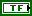freq in log? specifies whether to use logarithmic mapping for the frequency points. The default is TRUE.magnitude in dB? specifies whether to return the amplitude response in decibels. The default is TRUE.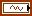stimulus signal specifies the input waveform of the stimulus signal.response signal specifies the input waveform of the response signal.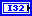window length specifies the length of the window to use in frequency computation. The value of window length must be less than the length of stimulus signal and greater than 0.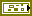error in describes error conditions that occur before this node runs. This input provides standard error in functionality.num of freq bins specifies the number of frequency bins for which this VI computes the frequency response. The value of num of freq bins must be greater than or equal to 2.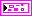magnitude response returns the amplitude response of an unknown system at a given frequency.freq point (mag) returns the frequency points at which this VI estimates the magnitude response.magnitude returns the estimated amplitude response at each freq point (mag).phase response returns the phase response of an unknown system at a given frequency.freq point (phase) returns the frequency points at which this VI estimates the phase response.phase returns the estimated phase response at each freq point (phase).std deviations returns the standard deviation for each estimated amplitude and phase response.magnitude std dev returns the standard deviation for each estimated amplitude response.phase std dev returns the standard deviation for each estimated phase response.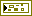error out contains error information. This output provides standard error out functionality.

## SI Estimate Frequency Response (Array)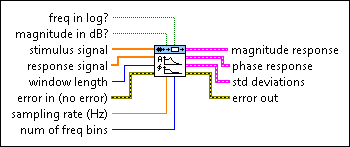freq in log? specifies whether to use logarithmic mapping for the frequency points. The default is TRUE.magnitude in dB? specifies whether to return the amplitude response in decibels. The default is TRUE.stimulus signal specifies an array that represents the stimulus signal.response signal specifies an array that represents the response signal.window length specifies the length of the window to use in frequency computation. The value of window length must be less than the length of stimulus signal and greater than 0.error in describes error conditions that occur before this node runs. This input provides standard error in functionality.sampling rate (Hz) specifies the sampling frequency in hertz. The value of sampling rate must be greater than 0. The default is 1.num of freq bins specifies the number of frequency bins for which this VI computes the frequency response. The value of num of freq bins must be greater than or equal to 2.magnitude response returns the amplitude response of an unknown system at a given frequency.freq point (mag) returns the frequency points at which this VI estimates the magnitude response.magnitude returns the estimated amplitude response at each freq point (mag).phase response returns the phase response of an unknown system at a given frequency.freq point (phase) returns the frequency points at which this VI estimates the phase response.phase returns the estimated phase response at each freq point (phase).std deviations returns the standard deviation for each estimated amplitude and phase response.magnitude std dev returns the standard deviation for each estimated amplitude response.phase std dev returns the standard deviation for each estimated phase response.error out contains error information. This output provides standard error out functionality.

## SI Estimate Frequency Response Details

The following equation defines a frequency response estimated using the spectral analysis method: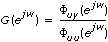where G(ejw) is the frequency response of the system.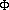uy(ejw) is the cross-spectral density between the stimulus signal and the response signal. This VI computesuy(ejw) by applying a Fourier transform on the cross correlation between the stimulus signal and the response signal.uu(ejw) is the auto-spectral density of the stimulus signal. This VI computesuu(ejw) by applying a Fourier transform on the autocorrelation of the stimulus signal.

In order to reduce leakage and improve resolution, this VI applies a Hanning window on the cross correlation and autocorrelation before applying the Fourier transform. The larger the window length, the larger the variance, but the smaller the bias of the estimated frequency response.

## Examples

Refer to the following VIs for examples of using the SI Estimate Frequency Response VI:

• Estimate Frequency Response VI: labview\examples\System Identification\Getting Started\Nonparametric Estimation.llb
• Steel Subframe Flexible Structure VI: labview\examples\System Identification\Industry Applications\Mechanical Systems.llb